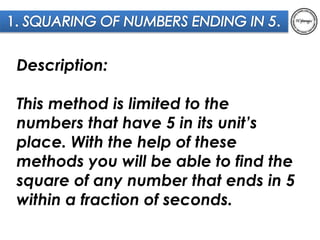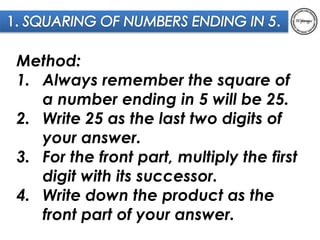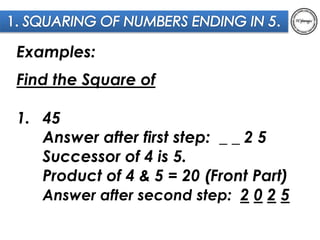Diese Präsentation wurde erfolgreich gemeldet.

# Square of Numbers that end in 5

Anzeige
Anzeige
Anzeige
Anzeige
Anzeige
Anzeige
Anzeige
Anzeige
Anzeige
Anzeige
Anzeige×

1 von 6 Anzeige

# Square of Numbers that end in 5

This method is limited to the numbers that have 5 in its unit’s place. With the help of these methods you will be able to find the square of any number that ends in 5 within a fraction of seconds.

This method is limited to the numbers that have 5 in its unit’s place. With the help of these methods you will be able to find the square of any number that ends in 5 within a fraction of seconds.

Anzeige
Anzeige

### Square of Numbers that end in 5

1. 1. Description: This method is limited to the numbers that have 5 in its unit’s place. With the help of these methods you will be able to find the square of any number that ends in 5 within a fraction of seconds.
2. 2. Method: 1. Always remember the square of a number ending in 5 will be 25. 2. Write 25 as the last two digits of your answer. 3. For the front part, multiply the first digit with its successor. 4. Write down the product as the front part of your answer.
3. 3. Examples: Find the Square of 1. 45 Answer after first step: _ _ 2 5 Successor of 4 is 5. Product of 4 & 5 = 20 (Front Part) Answer after second step: 2 0 2 5
4. 4. Examples: Find the Square of 1. 85 Answer after first step: _ _ 2 5 Successor of 8 is 9. Product of 8 & 9 = 72 (Front Part) Answer after second step: 7 2 2 5
5. 5. Examples: Find the Square of 1. 125 Answer after first step: _ _ _ 2 5 Successor of 12 is 13. Product of 12 & 13 = 156(Front Part) Answer after second step: 1 5 6 2 5
6. 6. Thank you